## Access NCERT Solutions for Class 6 Maths Chapter 14: Practical Geometry Exercise 14.2

### Access NCERT Solutions for Class 6 Maths Chapter 14: Practical Geometry Exercise 14.2

1. Draw a line segment of length 7.3 cm using a ruler.

Solutions:

Using a ruler, we can draw a line segment of length 7.3 cm as follows

Step 1: Mark a point A on the sheet

Step 2: Place 0 mark of ruler at point A

Step 3: At 7.3 cm on ruler, mark a point B on the sheetStep 4: Now join A and B

Here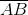is the required line segment.2. Construct a line segment of length 5.6 cm using ruler and compasses.

Solutions:

By using a ruler and compasses, we may draw a line segment of length 5.6 cm as follows

Step 1: Draw a line l and mark a point A on this line l.Step 2: On the zero mark of the ruler, place the compasses. Now extend the compasses to place the pencil up to the 5.6 cm and mark.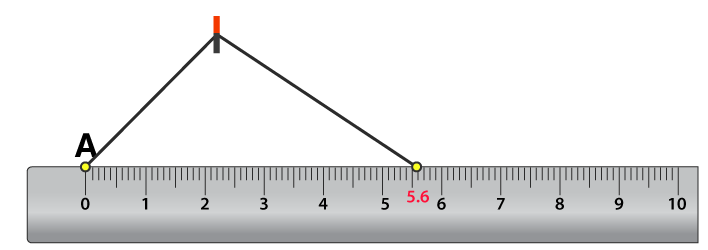Step 3: Place the pointer of compasses on point A and draw an arc to cut l at B. Now,is the line segment of 5.6 cm length.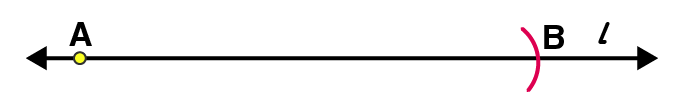3. Constructof length 7.8 cm. From this, cut offof length 4.7 cm. Measure.

Solutions:

Step 1: Draw a line l and mark a point A on it.Step 2: By adjusting the compasses up to 7.8 cm, while putting the pointer of compasses on point A draw an arc to cut l on Bis the line segment of 7.8 cmStep 3: By adjusting the compasses up to 4.7 cm, draw an arc to cut l on C, while putting the pointer of compasses on point A.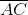is the line segment of of 4.7 cmStep 4: Now, place the ruler in such a way that 0 mark of the ruler will match with the point C.Now read the position of point B. It will be 3.1 cm.is 3.1 cm

4. Givenof length 3.9 cm, construct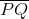such that the length ofis twice that of. Verify by measurement.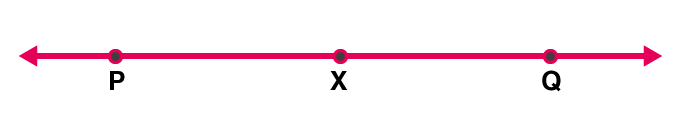Solutions:

We can draw a line segmentsuch that the length ofis twice that ofas follows

(1) Draw a line l and mark a point P on it. Let AB be the given line segment of 3.9 cm.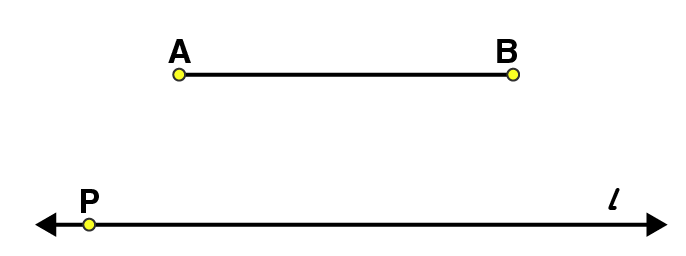(2) By adjusting the compasses up to the length of AB, place the pointer of compasses at point P and draw an arc to cut the line at X.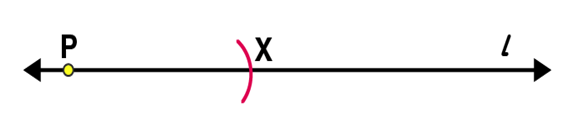(3) Again place the pointer on point X, draw an arc to cut line l at point Q.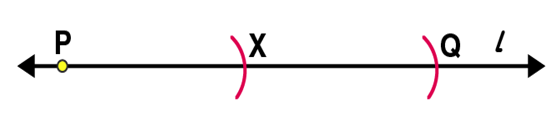is the required line segment. Using the ruler we may measure the length ofwhich comes to 7.8 cm.

5. Givenof length 7.3 cm and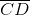of length 3.4 cm, construct a line segmentsuch that the length ofis equal to the difference between the lengths ofand. Verify by measurement.

Solutions:

(1) Given= 7.3 cm and= 3.4 cm(2) Adjust the compasses up to the length of CD and put the pointer of compasses at point A, draw an arc to cut AB at point P.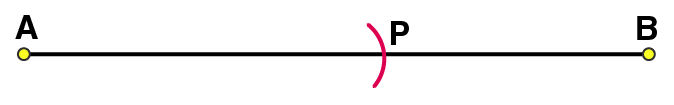(3) Adjust the compasses up to the length of PB. Draw a line l and mark a point X on it.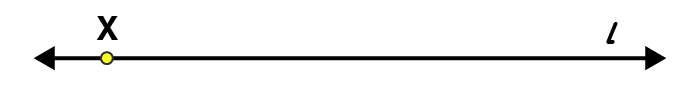(4) By placing the pointer of compasses at point X, draw an arc to cut the line at Y.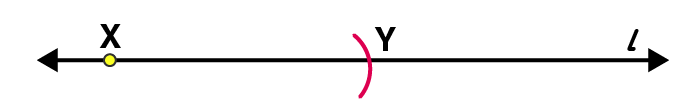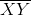is the required line segment

Now, difference between the lengths ofand= 7.3 – 3.4

= 3.9 cm

Using the ruler we may measure the length ofwhich comes to 3.9 cm.